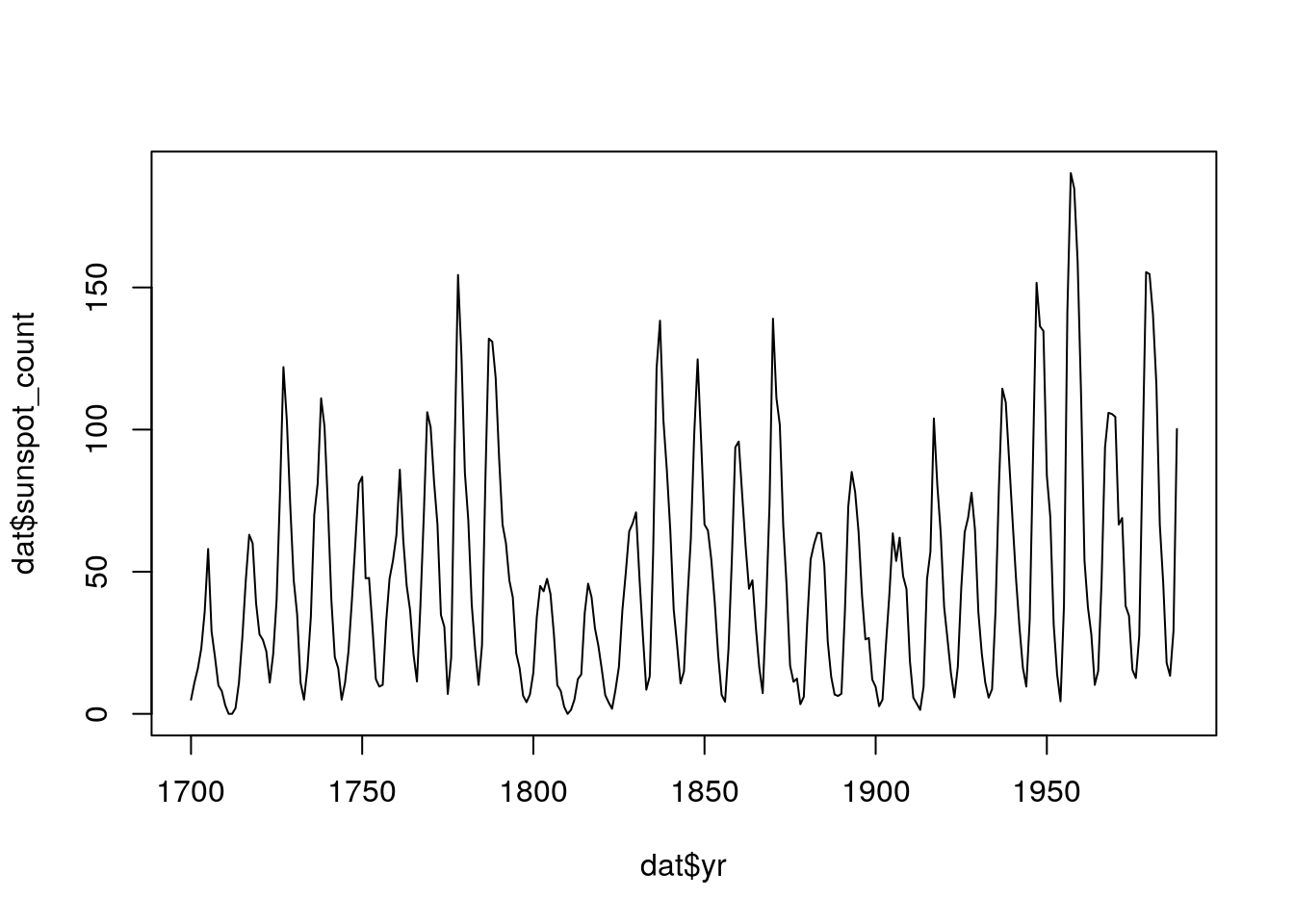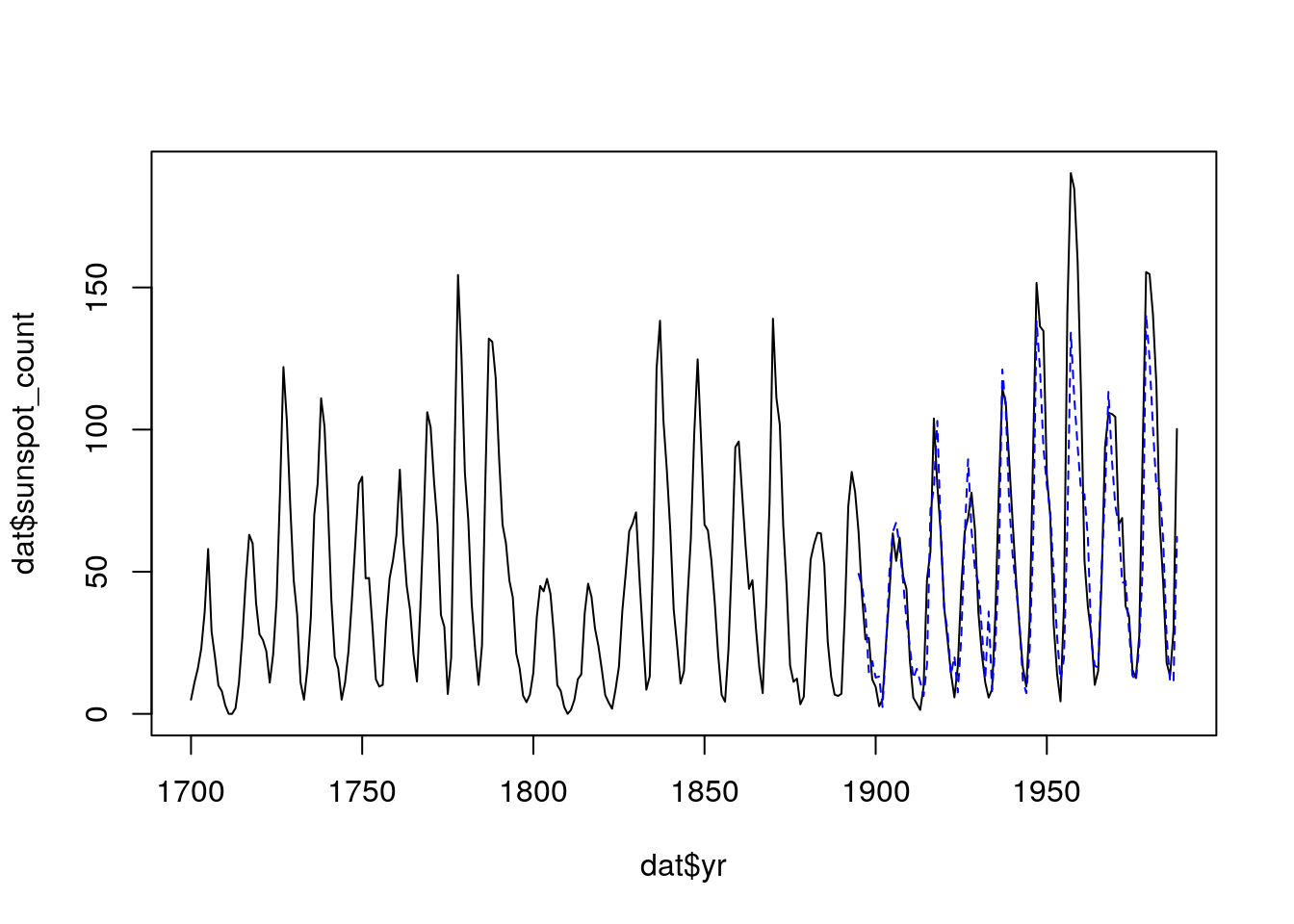# 1 Abstract

This document is designed to be a quick intro for “empiricial dynamic modeling” (EDM) and the rEDM package. For more detailed information, please see the rEDM-tutorial vignette include in the package [link].

# 2 Theory

Suppose that we have a system with $$d$$ state variables: $$x_1, x_2, \dots, x_d$$. We can represent the state at a specific time, $$t$$, as a vector with these $$d$$ components: $$\bar{x}(t) = \langle x_1(t), x_2(t), \dots, x_d(t) \rangle$$.

We generally assume that the system is deterministic, such that the current state fully determines future states: $\bar{x}(t+1) = F \left(\bar{x}(t)\right)$ though we may not know what this function $$F$$ actually is.

## 2.1 Example

In model systems, we usually know what $$F$$ is, since we have differential or difference equations that describe the system behavior. For example, the coupled logistic map equations are: \begin{align*} x_1(t+1) &= r_1 x_1(t) \left[1 - x_1(t) - \alpha_{1,2} x_2(t)\right]\\ x_2(t+1) &= r_2 x_2(t) \left[1 - x_2(t) - \alpha_{2,1} x_1(t)\right] \end{align*} with parameters $$r_1, r_2, \alpha_{1,2}, \alpha_{2,1}$$.

## 2.2 Time Delay Embedding (Delay Coordinate Embedding)

We would naturally expect that to predict the future of any component of the system, e.g. $$x_i$$, we would need to know all the other $$x_j$$ values. Takens’ Theorem actually posits that this is not strictly necessary, and that under certain conditions, a single time series is sufficient.

In other words, instead of relying on: $x_i(t+1) = F_i\left(x_1(t), x_2(t), \dots, x_d(t)\right)$

the system dynamics can be represented as a function of a single variable and its lags: $x_i(t+1) = G_i\left(x_i(t), x_i(t-1), \dots, x_i(t-(E-1))\right)$

Note that we use $$E$$ coordinates instead of $$d$$ and that we do not make any claims about how the functions $$F_i$$ and $$G_i$$ are related.

## 2.3 Usage

Since we generally don’t know the form of $$G_i$$, we stick to inferring it from the data. The rEDM package provides several methods to do so, but here we just stick to simplex projection.

Simplex projection uses a weighted nearest-neighbors approximation to estimate $$G_i$$. That’s kind of a fancy way of saying the following:

1. Suppose we have the value of $$x$$ and its lags at time $$s$$. Then we want a prediction of $$x(s+1) = G\left(x(s), x(s-1), \dots, x(s - (E-1))\right)$$.

2. We look for $$j = 1..k$$ nearest neighbors in the observed time series of $$x$$ such that $$\langle x(s), x(s-1), \dots, x(s - (E-1))\rangle \approx \langle x(n_j), x(n_j-1), \dots, x(n_j - (E-1))\rangle$$.

3. We then suppose that $$x(s+1) \approx x(n_j+1)$$.

Mathematically, this occurs by using some distance function to judge how similar $$\langle x(s), x(s-1), \dots, x(s - (E-1))\rangle$$ is to $$\langle x(n_j), x(n_j-1), \dots, x(n_j - (E-1))\rangle$$ and estimating $$x(s+1)$$ as a weighted average of the $$x(n_j+1)$$ values with weighting determined by the distances.

# 3 Applications

These principles of EDM can be used for several different applications. These include:

• univariate forecasting (predicting future behavior using lags of a single time series)
• multivariate forecasting (predicting future behavior using lags of multiple time series)
• characterizing various properties of time series (e.g. predictability and “nonlinearity”)
• identifying causal relationships between time series

Here, we’ll just look at univariate forecasting, though there are examples for the others among the package documentation. (see the vignettes on CRAN)

# 4 Univariate Forecasting

## 4.1 Data

We’ll start by looking at sunspot data. Note that there is also monthly data, but it seems quite a bit noisier:

dat <- data.frame(yr = as.numeric(time(sunspot.year)),
sunspot_count = as.numeric(sunspot.year))

plot(dat$yr, dat$sunspot_count, type = "l")## 4.2 Determining E

From the above sections, you may recall that one of the parameters is the embedding dimension, E. Since we ultimately want to test forecast skill, we use only the first 2/3 of the data to fit E. There are several other parameters that we can set, but the defaults work fine for our case:

library(rEDM)                    # load the package

n <- NROW(dat)
lib <- c(1, floor(2/3 * n))      # indices for the first 2/3 of the time series
pred <- c(floor(2/3 * n) + 1, n) # indices for the final 1/3 of the time series

simplex(dat,                     # input data (for data.frames, uses 2nd column)
lib = lib, pred = lib,   # which portions of the data to train and predict
E = 1:10)                # embedding dimensions to try
## Warning in model$run(): Found overlap between lib and pred. Enabling cross- ## validation with exclusion radius = 0. ## E tau tp nn num_pred rho mae rmse perc p_val ## 1 1 1 1 2 191 0.6991334 19.02584 26.30722 1 8.621117e-33 ## 2 2 1 1 3 190 0.9074985 10.73279 14.73790 1 2.037040e-95 ## 3 3 1 1 4 189 0.9195079 10.16759 13.93505 1 4.910681e-104 ## 4 4 1 1 5 188 0.9195163 10.46335 14.00166 1 1.703957e-103 ## 5 5 1 1 6 187 0.9176281 10.92784 14.17733 1 2.032115e-101 ## 6 6 1 1 7 186 0.9160703 11.21311 14.28820 1 1.170578e-99 ## 7 7 1 1 8 185 0.9082384 11.42164 14.98126 1 1.974748e-93 ## 8 8 1 1 9 184 0.8911547 11.98804 16.38659 1 1.675719e-82 ## 9 9 1 1 10 183 0.8708673 12.95866 17.75854 1 3.249362e-72 ## 10 10 1 1 11 182 0.8561815 13.38768 18.57338 1 6.248548e-66 ## const_pred_num_pred const_pred_rho const_pred_mae const_pred_rmse ## 1 191 0.8181284 16.30681 21.10918 ## 2 190 0.8171769 16.36105 21.16018 ## 3 189 0.8164772 16.42116 21.21297 ## 4 188 0.8160786 16.47128 21.26318 ## 5 187 0.8162301 16.48984 21.29875 ## 6 186 0.8171832 16.46022 21.29492 ## 7 185 0.8188354 16.39243 21.24568 ## 8 184 0.8186807 16.43261 21.29300 ## 9 183 0.8181869 16.46776 21.33830 ## 10 182 0.8171689 16.54725 21.39633 ## const_pred_perc const_p_val ## 1 1 2.020297e-56 ## 2 1 7.301909e-56 ## 3 1 2.220877e-55 ## 4 1 5.537661e-55 ## 5 1 9.715865e-55 ## 6 1 1.027021e-54 ## 7 1 6.966389e-55 ## 8 1 1.498706e-54 ## 9 1 3.987699e-54 ## 10 1 1.463741e-53 The output is a data.frame describing how well the model “predicted” on the given data. The columns are various statistical outputs. We’ll focus just on the measures of model performance: • rho (correlation between observed and predicted values) • mae (mean absolute error) • rmse (root mean squared error) It looks like E = 3 or 4 is optimal. Since we generally want a simpler model, if possible, let’s go with E = 3 to forecast the remaining 1/3 of the data. ## 4.3 Forecasts We can use similar code to produce forecasts for the last 1/3 of the time series. Since we want the outputs in addition to the performance statistics, we add an additional argument to the function call: output <- simplex(dat, lib = lib, pred = pred, # predict on last 1/3 E = 3, stats_only = FALSE) # return predictions, too Here the output is again a data.frame with a list column for the predictions: predictions <- output$model_output[]
str(predictions)
## 'data.frame':    97 obs. of  4 variables:
##  $time : num 1893 1894 1895 1896 1897 ... ##$ obs     : num  85.1 78 64 41.8 26.2 26.7 12.1 9.5 2.7 5 ...
##  $pred : num NaN NaN 49.3 45 36.2 ... ##$ pred_var: num  NaN NaN 24 40.6 88.2 ...

Let’s plot the predictions against the original data:

plot(dat$yr, dat$sunspot_count, type = "l")
lines(predictions$time, predictions$pred, col = "blue", lty = 2)Since we also have an estimate of the prediction uncertainty, let’s include that as well:

plot(dat$yr, dat$sunspot_count, type = "l")
lines(predictions$time, predictions$pred, col = "blue", lty = 2)
polygon(c(predictions$time, rev(predictions$time)),
c(predictions$pred - sqrt(predictions$pred_var),
rev(predictions$pred + sqrt(predictions$pred_var))),
col = rgb(0, 0, 1, 0.5), border = NA)